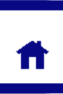# Temperature and Equilibrium.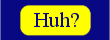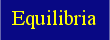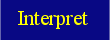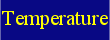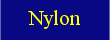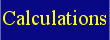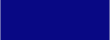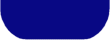Students learn to identify that temperature is the only factor that changes the value of the equilibrium constant (K).

The equilibrium constant K will always have a constant value for any given temperature. However if the temperature of a system is changed then the value of K will also change.

An example of this is seen with the following system: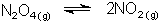The expression for K is as follows: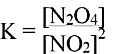Temperature dependence for K is seen when K is evaluated at 25 and 100°C.

 Temperature (°) K 25 0.15 100 15.0